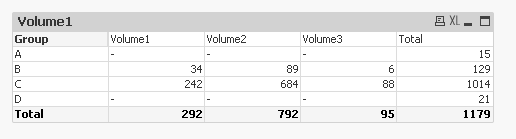# QlikView App Dev

Discussion Board for collaboration related to QlikView App Development.

cancel
Showing results for
Did you mean:Creator

## how to block data?

Hi

any suggestions on how to block this data when total drop 25?

chart type is pivot table. I want to show hypen in vol 1,2,3 if the row total is less then 25 as highlighted in example below2 RepliesCreator III

i would calculate like this

IF( AGGR( SUM(VALUE),GROUP)<25,NUll(),SUM(VALUE))Champion III

Another way can be tweaking a little of Chaitanya expr Try like:

Dim: Group

Expr:

= IF(Aggr(Rangesum(Vol1, Vol2, Vol3), Group) < 25, '-', Sum(Vol1))

= IF(Aggr(Rangesum(Vol1, Vol2, Vol3), Group) < 25, '-', Sum(Vol2))

= IF(Aggr(Rangesum(Vol1, Vol2, Vol3), Group) < 25, '-', Sum(Vol3))

= Sum(Aggr(Rangesum(Vol1, Vol2, Vol3), Group))

Last expr is for Totals.

Below is how it looksCommunity Browser